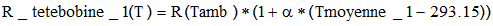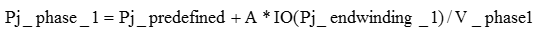# Taking into consideration the losses in the motors end winding in 2D

## Presentation

The losses by Joule effect in the parts of coils outside the magnetic cores or end winding represent an important part of the total coils Joule losses: they can reach 25% of the total Joule losses in the winding. The consideration of these end winding is therefore essential, also in 2D project where user can't represent directly end winding geometry.

## Losses in the end winding vs cosimulation

The method applied in the cosimulation permits the calculation and export of the values on the mesh nodes. Consideration of the losses in the end winding, represented by resistors in the circuit model, within the cosimulation, must therefore be done in a bypass manner (current limitation).

The general principle consists of:

• Averaging the temperature imported in the electromagnetic problem in order to take into account an average temperature in the evolution of the value of winding resistance
• Distributing the calculated Joule losses in the resistors that model the end winding over the multipoint supports containing the thermal mesh

## Distribution of the Joule losses of the end winding

Concerning the last point mentioned in the previous section, the distribution of the Joule losses of the end winding is done manually. The user is free to choose the distribution he wishes.

In general, it is advisable to distribute them on:

• the conductors (around the 2/3 level, for example)
• the stator teeth: the proximity of the end winding heats that part (around the 1/3 level, for example)

## Steps

The steps to follow in the electromagnetic project in order to take into consideration the Joule losses in the end winding in the cosimulation are presented below.

Step Action
1

Create sensors to calculate the average temperature starting from the imported temperatures (for each phase of the winding):

• of the servo sensor type (evaluated at the beginning of steps)
• of the integral type: choose the regions of winding of one phase
• with a spatial formula « TKelvin/Surface of the regions»
• with an integration in the plane

We will name «Tmean_1» the averaged temperature on the phase 1

2

Define the resistances of the end winding by the expression depending on the temperature:Troom : ambient temperature

3

Create an I/O parameter to define the Joule losses in the end winding (R*I²) for each phase (use the calculator to choose R and I)

We will name « Pj_end winding_1» the Joule losses in the coil overhangs of phase 1

4

In the multiphysics context, create multiphysics formulas to take into consideration the Joule losses of the end winding. For example :

• Joule losses in the phase 1:Pj_predefined : predefined multiphysics formula “joule losses”

A : < 1, ratio of distribution of the end winding losses

V_phase1 : volume of the region associated to the phase 1

IO() : this command (non accessible in the calculator) permits the taking into consideration of an I/O parameter.